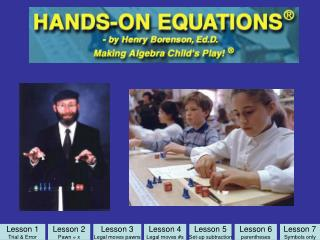DownloadDownload PresentationLesson 2 Pawn = x

# Lesson 2 Pawn = x

Télécharger la présentation## Lesson 2 Pawn = x

- - - - - - - - - - - - - - - - - - - - - - - - - - - E N D - - - - - - - - - - - - - - - - - - - - - - - - - - -
##### Presentation Transcript

1. Lesson 1 Trial & Error Lesson 2 Pawn = x Lesson 3 Legal moves pawns Lesson 4 Legal moves #s Lesson 5 Set-up subtraction Lesson 6 parentheses Lesson 7 Symbols only

2. Comparing Earth and Moon Mass Equal and Balanced

3. Equal and Balanced 100 pounds = ? pounds

4. Materials for Book 1 • Eight blue pawns • Two red cubes, numbered 0-5 • Two red cubes, numbered 5-10 • A laminated balance scale • Put the other items back in the bag.

5. What is the value for the blue pawn to balance the scale? 5 5 5 Check: = X = 5 5 5 Lesson 1

6. Use trial and error to solve. 8 4 + 4 8 8 = Check: 8 8 X = 4 Lesson 1

7. Use trial and error method to solve. 1, 2, 3, etc. 2 8 Check: 14 14 = X = 6 The blue pawn has a special name, “x”. Lesson 1

8. Please place number cubes facing upward so I can see the numbers when I pass by. Black triangles are symbols for blue pawns 10 Check: X = Lesson 1

9. 2 9 7 + 2 9 9 Check: = X = 7 9 9 Lesson 1

10. 3 7 2+2 + 3 7 7 Check: = X = 2 7 7 Lesson 1

11. 8 4 + 8 4+4+4 12 12 Check: = X = 4 12 12 Lesson 1

12. 2 4 1 + 1 + 2 4 4 Check: = X = 1 4 4 Lesson 1

13. 8 10 4 6 + 8 10 +4 14 14 Check: = X = 6 14 14 Lesson 1

14. 4 4 + 4 4 + 4 8 8 Check: = X = 4 8 8 Lesson 1

15. 2 7 7 4+4+4 + 2 7 + 7 14 14 Check: = X = 4 14 14 Lesson 1

16. 4 10 6+6 + 4 6 + 10 16 16 Check: = X = 6 16 16 Lesson 1

17. 10 2 6+6 +6 6+10+ 2 18 18 Check: = X = 6 18 18 Lesson 1

18. Lesson 1 Trial & Error Lesson 2 Pawn = x Lesson 3 Legal moves pawns Lesson 4 Legal moves #s Lesson 5 Set-up subtraction Lesson 6 parentheses Lesson 7 Symbols only

19. 8 The pawn has a special name, “x.” Therefore, the problem 2x + x = x + 8 2 Check: 12 12 = X = 4 Lesson 2

20. 1 7 3x + 1 = x + 7 Check: 10 10 = X = 3 Lesson 2

21. 3 1. 2x = x + 3 Check: 6 6 = X = 3 Lesson 2

22. 2. 3x = x + 4 4 6 6 = Check: X = 2 Lesson 2

23. 3. x + 4 = 2x + 3 4 3 5 5 = Check: X = 1 Lesson 2

24. 4. 4x = 2x + 6 6 12 12 = Check: X = 3 Lesson 2

25. Lesson 1 Trial & Error Lesson 2 Pawn = x Lesson 3 Legal moves pawns Lesson 4 Legal moves #s Lesson 5 Set-up subtraction Lesson 6 parentheses Lesson 7 Symbols only

26. Lesson 3 • Try this one. • 4x + 2 = 3x + 9 • “Would you like to learn an easier way of getting the answer than by using trial and error?”

27. 4x + 2 = 3x + 9 2 9 Legal Moves X = 7 Lesson 3

28. Reset the original equation to check. 4x + 2 = 3x + 9 2 9 Check: 30 30 = X = 7 Lesson 3

29. 1. 3x + 7 = 4x Set up X = Lesson 3

30. 1. 3x + 7 = 4x 7 Legal Moves X = 7 Lesson 3

31. 1. 3x + 7 = 4x 7 Check: 28 28 = X = 7 Lesson 3

32. 2. x + 2 +2x = x + 10 Set up X = Lesson 3

33. 2. x + 2 +2x = x + 10 2 10 Legal Moves X = 4 Lesson 3

34. 2. x + 2 +2x = x + 10 2 10 Check: 14 14 = X = 4 Lesson 3

35. 3. x + 3x = x + x + 10 Set up X = Lesson 3

36. 3. x + 3x = x + x + 10 10 Legal Moves X = 5 Lesson 3

37. 3. x + 3x = x + x + 10 10 Check: 20 20 = X = 5 Lesson 3

38. 4. 2x + 3 + 3x = x + 11 Set up X = Lesson 3

39. 4. 2x + 3 + 3x = x + 11 3 10 1 Legal Moves X = 2 Lesson 3

40. 4. 2x + 3 + 3x = x + 11 3 10 1 Check: 13 13 = X = 2 Lesson 3

41. Lesson 1 Trial & Error Lesson 2 Pawn = x Lesson 3 Legal moves pawns Lesson 4 Legal moves #s Lesson 5 Set-up subtraction Lesson 6 parentheses Lesson 7 Symbols only

42. 1. x + 2x +5 = x + 19 Set up X = Lesson 4